# 图像处理中的边缘密度

## 浅谈安防监控中视频图像处理技术

2019-08-16 08:48:45 weixin_42137700 阅读数 939
• ###### MATLAB图像处理

全面系统的学习MATLAB在图像处理中的应用

56课时 487分钟 21233人学习 魏伟
免费试看

2019-08-15 21:39:20

1、将(运动)目标从视频图像中分离出来。2、对目标进行行为分析，判定其运动的方向、方式，并能发现和告警异常的行为；产生目标的运动轨迹，并能进行目标的自动跟踪。3、实现视频语义的解析，图像处理分析的高层次。通过对一个图像序列作出分析，得出其包含的真实信息，可以与话音的语义解析结合起来，逐步实现视频语义的解析。4、在复杂环境下实现目标的分离、行为分析和运动跟踪，特别是实现多目标的跟踪。

## 图像处理与识别学习小结

2009-07-23 22:30:00 byxdaz 阅读数 23744
• ###### MATLAB图像处理

全面系统的学习MATLAB在图像处理中的应用

56课时 487分钟 21233人学习 魏伟
免费试看

RGB (red green blue): 红绿蓝三基色

CMYK (Cyan-Magenta-Yellow-black inK): 青色-品红-黄色-黑色

HSI (Hue-Saturation-Intensity): 色调-饱和度-强度

DDB (device-dependent bitmap): 设备相关位图

DIB (device-independent bitmap): 设备无关位图

CVBS (Composite Video Broadcast Signal): 复合电视广播信号

YUV（亦称Y Cr Cb）是被欧洲电视系统所采用的一种颜色编码方法（属于PAL制）。Png格式、swf格式、svg格式、pcx格式、dxf格式、wmf格式、emf格式、LIC格式、eps格式、TGA格式。

OpenCV源代码及文档下载：SOURCEFORGE.NET
http://sourceforge.net/projects/opencvlibrary/

A空间域增强处理

1）、空域变换增强

直方图均衡化基本做法是将每个灰度区间等概率分布代替了原来的随机分布，即增强后的图象中每一灰度级的像元数目大致相同。直方图均衡化可使得面积最大的地物细节得以增强，而面积小的地物与其灰度接近的地物进行合并，形成综合地物。减少灰度等级换取对比度的增大。

直方图归一化是把原图像的直方图变换为某种指定形态的直方图或某一参考图像的直方图，然后按着已知的指定形态的直方图调整原图像各像元的灰级，最后得到一个直方图匹配的图像。这种方法主要应用在有一幅很好的图像作为标准的情况下，对另一幅不满意的图像用标准图像的直方图进行匹配处理，以改善被处理图像的质量。如在数字镶嵌时，重叠区影像色调由于时相等原因差异往往很大，利用直方图匹配这一方法后可以改善重叠区影像色调过度，如果镶嵌图像时相相差不大，完全可以作到无缝镶嵌。

2）、空域滤波增强

1）梯度法

梯度反映了相邻像元的亮度变化率，即图像中如果存在边缘，如湖泊、河流的边界，山脉和道路等，则边缘处有较大的梯度值。对于亮度值较平滑的部分，亮度梯度值较小。因此，找到梯度较大的位置，也就找到边缘，然后再用不同的梯度计算值代替边缘处像元的值，也就突出了边缘，实现了图像的锐化。通常有罗伯特梯度和索伯尔梯度方法。

2）拉普拉斯算法

拉普拉斯算法的意义与梯度法不同，它不检测均匀的亮度变化，而是检测变化率的变化率，相当于二阶微分。计算出的图像更加突出亮度值突变的位置。

3）定向检测

当有目的地检测某一方向的边、线或纹理特征时，可选择特定的模板卷积运算作定向检测。可以检测垂直边界、水平边界和对角线边界等，各使用的模板不同

B频率域图像增强处理1. 取函数h(m,n)关于原点的镜像，得到h(-m,-n)
2. 对某个(x,y),使h(-m,-n)移动相应的距离，得到h(x-m,y-n)
3. 对积函数f(m,n)h(x-m,y-n)(m,n)的取值范围内求和
4. 位移是整数增量，对所有的(x,y)重复上面的过程，直到两个函数：f(m,n)h(x-m,y-n)不再有重叠的部分。1. 对具有同样大小的空域和频率滤波器：h(x,y), H(u,v)，频域计算(由于FFT)往往更有效(尤其是图像尺寸比较大时）。但对在空域中用尺寸较小的模板就能解决的问题，则往往在空域中直接操作。

2. 频域滤波虽然更直接，但如果可以使用较小的滤波器，还是在空域计算为好。    因为省去了计算傅立叶变换及反变换等步骤。

3. 由于更多的直观性，频率滤波器设计往往作为空域滤波器设计的向导。

、理想低通滤波器
、巴特沃思低通滤波器
、高斯低通滤波器Roberts边缘检测算子

Sobel边缘算子

Prewitt边缘算子

Kirsch边缘算子

CANNY边缘检测

LZW算法

膨胀

A、阈值分割包括以下几种：

1）由直方图灰度分布选择阈值

2）双峰法选择阈值

3）迭代法选取阈值

原理如下，很好理解。

迭代法是基于逼近的思想，其步骤如下：
1． 求出图象的最大灰度值和最小灰度值，分别记为ZMAX和ZMIN，令初始阈值T0=(ZMAX+ZMIN)/2；
2． 根据阈值TK将图象分割为前景和背景，分别求出两者的平均灰度值ZO和ZB；
3． 求出新阈值TK+1=(ZO+ZB)/2；
4． 若TK=TK+1，则所得即为阈值；否则转2，迭代计算。

4 ）大津法选择阈值

5）由灰度拉伸选择阈值

B、区域的分割

区域生长算法

C基于形态学分水岭的分割

A 颜色特征

B 纹理特征

1）统计方法统计方法的典型代表是一种称为灰度共生矩阵的纹理特征分析方法Gotlieb Kreyszig 等人在研究共生矩阵中各种统计特征基础上，通过实验，得出灰度共生矩阵的四个关键特征：能量、惯量、熵和相关性。统计方法中另一种典型方法，则是从图像的自相关函数（即图像的能量谱函数）提取纹理特征，即通过对图像的能量谱函数的计算，提取纹理的粗细度及方向性等特征参数

2）几何法

3）模型法

4）信号处理法

：粗糙度、对比度、方向度、线像度、规整度和粗略度。自回归纹理模型（simultaneous auto-regressive, SAR）是马尔可夫随机场（MRF）模型的一种应用实例。

C形状特征

1）边界特征法该方法通过对边界特征的描述来获取图像的形状参数。其中Hough 变换检测平行直线方法和边界方向直方图方法是经典方法。Hough 变换是利用图像全局特性而将边缘像素连接起来组成区域封闭边界的一种方法，其基本思想是点—线的对偶性；边界方向直方图法首先微分图像求得图像边缘，然后，做出关于边缘大小和方向的直方图，通常的方法是构造图像灰度梯度方向矩阵。

2）傅里叶形状描述符法

3）几何参数法

D空间关系特征

## 图像处理之图像的边缘、轮廓检测

2017-06-15 10:20:10 llh_1178 阅读数 13061
• ###### MATLAB图像处理

全面系统的学习MATLAB在图像处理中的应用

56课时 487分钟 21233人学习 魏伟
免费试看

Canny函数中包含两个阈值这也是与其他边缘检测函数不同的地方。阈值是来确定哪里是边缘位置。阈值越低，能够检测出的边线越多，结果也就越容易受到图片噪声的影响，并且越容易从图像中挑出不相关的特性。与此相反，一个高的阈值将会遗失细的或者短的线段。因此，Canny函数中要设置两个阈值是有不同用处的。高的那个阈值是将要提取轮廓的物体与背景区分开来，就像阈值分割的那个参数一样，是决定目标与背景对比度的，低的那个阈值是用来平滑边缘的轮廓，有时高的阈值设置太大了，可能边缘轮廓不连续或者不够平滑，通过低阈值来平滑轮廓线，或者使不连续的部分连接起来。

```import cv2
from matplotlib import pyplot as plt
edges = cv2.Canny(img, 200, 300)
plt.subplot(1,2,1), plt.imshow(img,cmap = 'gray')
plt.title('Original Image'), plt.xticks([]), plt.yticks([])
plt.subplot(1,2,2), plt.imshow(edges,cmap = 'gray')
plt.title('Edge Image'), plt.xticks([]), plt.yticks([])
plt.show()``````import cv2
import numpy as np

img = np.zeros((200, 200), dtype=np.uint8)
img[50:150, 50:150] = 255
# 二值化处理
ret, th = cv2.threshold(img, 127, 255, 0)
# findcontours（）函数。有三个参数：输入图像、层次类型和轮廓逼近方法。
# 由函数返回的层次树相当重要：cv2.RETR_TREE参数会得到图像中轮廓的整体层次结构(contours)。如果只想得到最外面的轮廓，可以使用cv2.RETR_EXTERNAL。这对消除包含在其他轮廓中的轮廓很有用。
# 第三个参数有两种方法：
#cv2.CHAIN_APPROX_NONE存储所有的轮廓点，相邻的两个点的像素位置差不超过1，即max（abs（x1-x2），abs（y2-y1））==1 cv2.CHAIN_APPROX_SIMPLE压缩水平方向，垂直方向，对角线方向的元素，只保留该方向的终点坐标，例如一个矩形轮廓只需4个点来保存轮廓信息cv2.CHAIN_APPROX_TC89_L1，CV_CHAIN_APPROX_TC89_KCOS使用teh-Chinl chain 近似算法
image, contours, hierarchy = cv2.findContours(th, cv2.RETR_TREE, cv2.CHAIN_APPROX_SIMPLE)
# 色彩空间转换函数cv2.cvtColor()
color = cv2.cvtColor(img, cv2.COLOR_BAYER_BG2BGR)
# 画出轮廓，-1,表示所有轮廓，画笔颜色为(0, 255, 0)，即Green，粗细为2
img = cv2.drawContours(color, contours, -1, (0, 255, 0), 2)
cv2.imwrite("contours.png", color)
cv2.waitKey()
cv2.destroyAllWindows()```《OpenCV 3计算机视觉Python语言实现》

OpenCV帮助文档：http://docs.opencv.org/3.0.0/da/d22/tutorial_py_canny.html

## 关于图像处理中的卷积运算——sobel边缘检测

2019-08-27 17:20:20 weixin_43606771 阅读数 305
• ###### MATLAB图像处理

全面系统的学习MATLAB在图像处理中的应用

56课时 487分钟 21233人学习 魏伟
免费试看

## 一、卷积的概念以及计算

### 1.一维卷积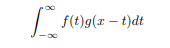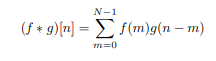### 2.二维卷积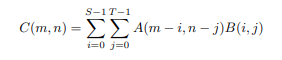## 二、图像处理中的应用

### 1.计算实例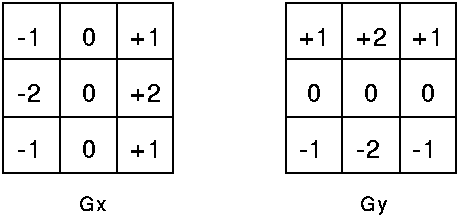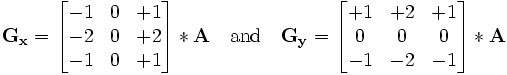### 2.方法汇总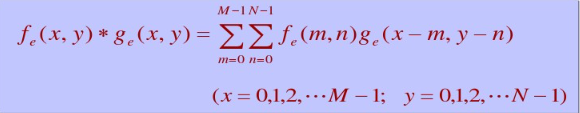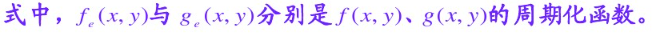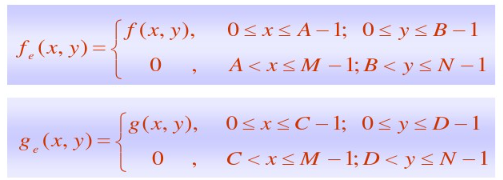A、B表示g(x,y)的维数，M、N代表f(x,y)的维数。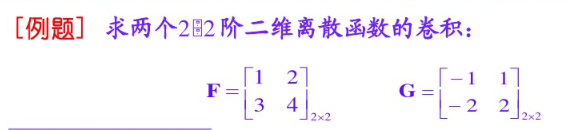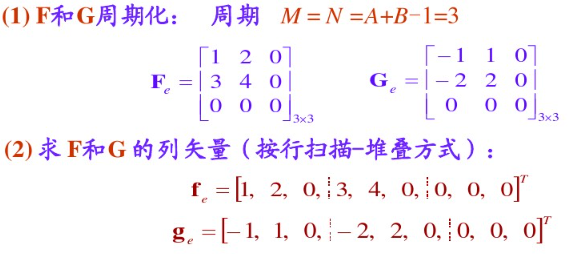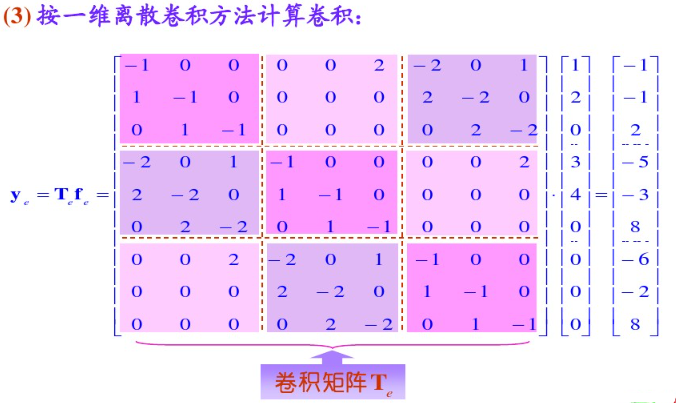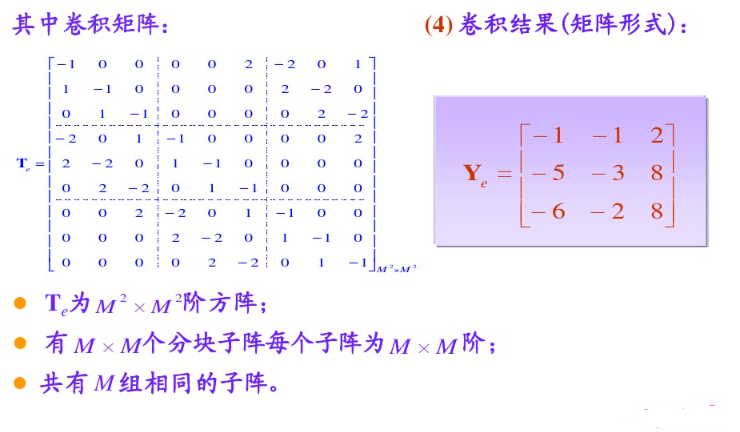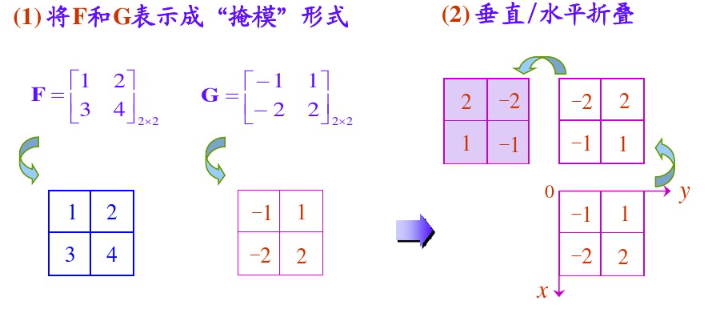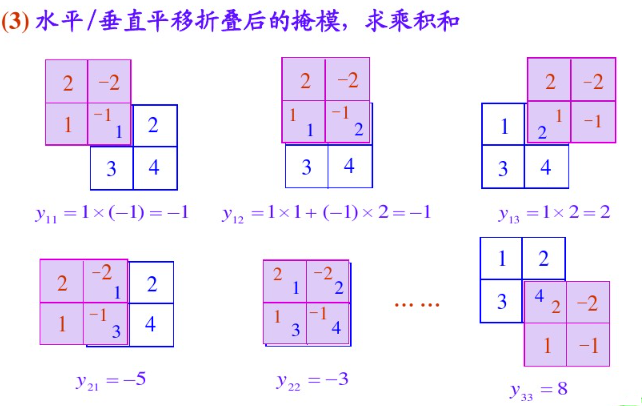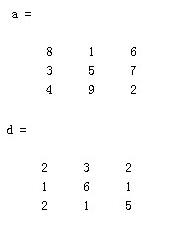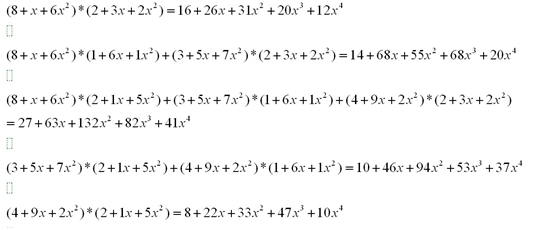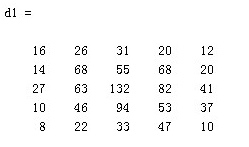B(0,0)=G(0,0)F(0,0)+G(0,1)F(0,-1)+G(1,0)F(-1,0)+G(1,1)F(-1,-1)最后代入数值，需要注意的是F为周期性函数，G为卷积核，F为被卷机矩阵，那么F应被扩展为（m+n）维的方阵，m、n分别为G、F的维数，且这个矩阵多余的补0，最终计算的式子其实与方法二相同。

### 3.计算结果

Gx =(-1)f(x-1, y-1) + 0f(x-1,y) + 1f(x-1,y+1) +(-2)f(x,y-1) + 0f(x,y)+2f(x,y+1) +(-1)f(x+1,y-1) + 0f(x+1,y) + 1f(x+1,y+1)= f(x-1,y+1)+2f(x,y+1)+f(x+1,y)-f(x-1, y-1)-2*f(x,y-1)-f(x+1,y-1)

Gy =1* f(x-1, y-1) + 2f(x-1,y)+ 1f(x,y+1) +0f(x,y-1)+ 0f(x,y) + 0*f(x,y+1) +(-1)f(x+1,y-1) + (-2)f(x+1,y-1) + (-1)f(x+1, y+1)= f(x-1, y-1) + 2f(x-1,y)+ 1f(x,y+1)-f(x+1,y-1)-2f(x+1,y-1) -f(x+1, y+1)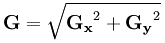## 三、MATLAB实现

``````f = imread('D:\GG\6c2a0008d4bf2b6df897.jpg');
f=rgb2gray(f);
n=input('请输入均值滤波模板大小：');
% tem=ones(n);
[hight,width]=size(f);
x1=double(f);x2=double(f);
for i=1:hight-n+1
for j=1:width-n+1
a=x1(i:i+n-1,j:j+n-1);
B =sort( a(:));
suma=sum((a(:)).^2);
x1(i+(n-1)/2,j+(n-1)/2)=sqrt(suma/(n*n));
x2(i+(n-1)/2,j+(n-1)/2)=median(B);
end
end
uSobel = f;
for i = 2:hight - 1   %sobel边缘检测
for j = 2:width - 1
Gx = (x1(i-1,j-1) - x1(i-1,j+1) + 2*x1(i,j-1)) - 2*x1(i,j+1) + x1(i+1,j-1) - x1(i+1,j+1);%不翻转
% Gx = (-x1(i-1,j-1) + x1(i-1,j+1) - 2*x1(i,j-1)) + 2*x1(i,j+1) - x1(i+1,j-1) + x1(i+1,j+1);%翻转
Gy = (-x1(i-1,j-1) - 2*x1(i-1,j) - x1(i-1,j+1)) +x1(i+1,j-1) + 2*x1(i+1,j) + x1(i+1,j+1);%不翻转
% Gy = (x1(i-1,j-1) + 2*x1(i-1,j) + x1(i-1,j+1)) -x1(i+1,j-1) - 2*x1(i+1,j) - x1(i+1,j+1);%翻转
uSobel(i,j) = sqrt(Gx^2 + Gy^2);
end
end
g = uint8(x1);
z = uint8(x2);
uSobel = uint8(uSobel);
subplot(1,4,1);
imshow(g);
title('均值滤波图像');
subplot(1,4,2);
imshow(f);
title('原图');
subplot(1,4,3);
imshow(z);
title('中值滤波图像');
subplot(1,4,4);
BW3 = edge(f,'Sobel', 0.02);
imshow(BW3);
title('边缘检测');
figure(2);
imshow(uSobel );
``````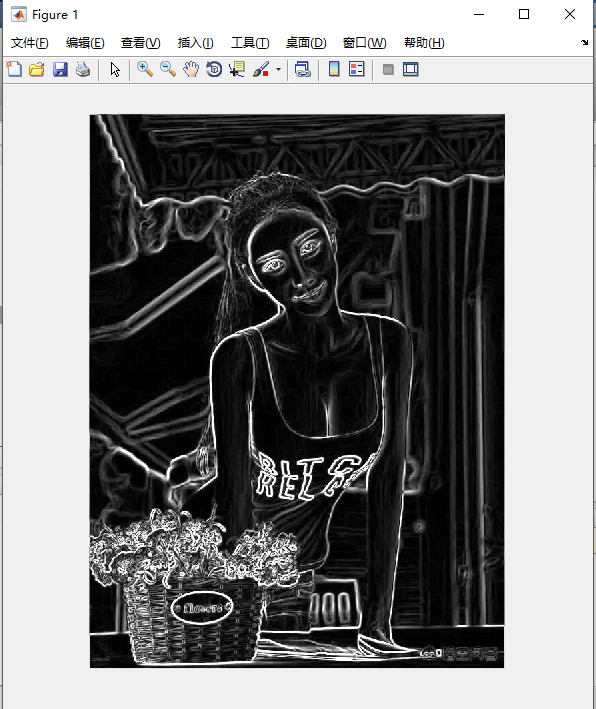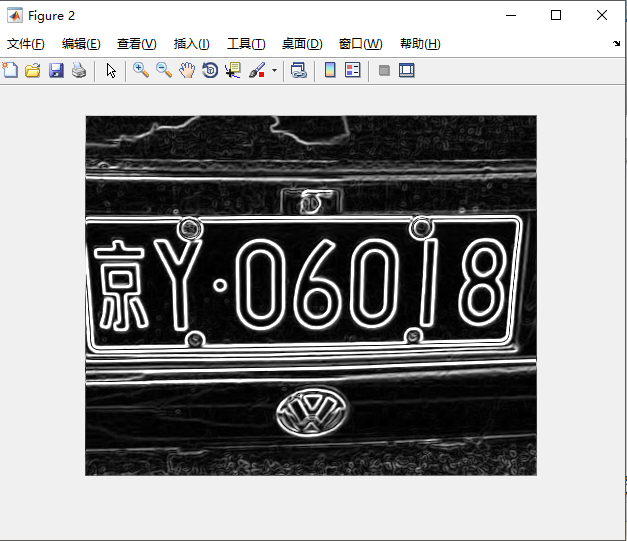1.卷积运算 Convolution (f ∗ g)(x)
2.sobel边缘检测

## 图像边缘检测与边缘增强处理——（Roberts、prewitt、sobel）

2018-04-07 19:09:19 IMWTJ123 阅读数 36443
• ###### MATLAB图像处理

全面系统的学习MATLAB在图像处理中的应用

56课时 487分钟 21233人学习 魏伟
免费试看

## 一、基本原理

### 1.1图像边缘

图像边缘是图像最基本的特征之一，往往携带着一幅图像的大部分信息。而边缘存在于图像的不规则结构和不平稳现象中，也即存在于信号的突变点处，这些点给出了图像轮廓的位置，这些轮廓常常是我们在图像边缘检测时所需要的非常重要的一些特征条件，这就需要我们对一幅图像检测并提取出它的边缘。而边缘检测算法则是图像边缘检测问题中经典技术难题之一，它的解决对于我们进行高层次的特征描述、识别和理解等有着重大的影响；又由于边缘检测在许多方面都有着非常重要的使用价值，所以人们一直在致力于研究和解决如何构造出具有良好性质及好的效果的边缘检测算子的问题。

### 1.2边缘检测的定义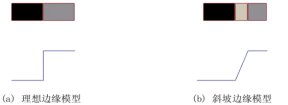一条理想的边缘应该具有如图(a)所示模型的特性。每个像素都处在灰度级跃变的一个垂直的台阶上（例如图形中所示的水平线通过图像的灰度剖面图）。而实际上，诸如图像采集系统的性能、采样频率和获得图像的照明条件等因素的影响，得到的边缘往往是模糊的，边缘被模拟成具有“斜坡面”的剖面，如图(b)所示，在这个模型中不再有细线（宽为一个像素的线条），而是出现了边缘的点包含斜坡中任意点的情况。由此可以看到：模糊的边缘使边缘的“宽度”较大，面清晰的边缘使边缘的宽度较小。图像的边缘有方向的幅度两个属性，沿边缘方向像素变化平缓，垂直于边缘方向像素变化剧烈。边缘上的这种变化可以用微分算子检测出来，通常用一阶导数或二阶导数来检测边缘，不同的是一阶导数认为最大值对应边缘位置，而二阶导数以过零点对应边缘位置。实际上，对于图像中的任意方向上的边缘都可以进行类似的分析。图像边 缘检测中对任意点的一阶导数可以利用该点梯度的幅度来获得，二阶导数可以用拉普拉斯算子得到。

### 1.3图像边缘检测算法的研究内容

图像边缘检测和分析可定义为应用一系列方法获取、校正、增强、变换、检测或压缩可视图像的技术。其目的是提高信息的相对质量，以便提取有用信息。图像边缘检测中的变换属于图像输入-图像输出模式，图像边缘检测是一种超越具体应用的过程，任何为解决某一特殊问题而开发的图像边缘检测新技术或新方法，几乎肯定都能找到其他完全不同的应用领域。图像边缘检测的主要研究内容包括：
（1）图像获得和抽样，其中通过人眼观察的视野获取图像的问题有：最常用的图像获取装置——电视（TV）摄像机问题，对所获得信号进行独立的采样和数字化就可用数字形式表达景物中全部彩色内容；电荷-耦合装置，用作图像传感器，对景物每次扫描一行，或通过平行扫描获得图像；选择正确的分辨力或采样密度，一幅图像实质上是二维空间中的信号，所以适用于信号处理的法则同样适用于图像边缘检测，在放射学中常常需要高分辨力，要求图像至少达到2048像素×2048像素；灰度量化，图像强度也必须进行数字化，通常以256级（按1字节编码）覆盖整个灰度，一般一幅灰度分辨力为8位，空间分辨力为512像素×512像素的图像需0.25兆字节的存贮容量。
（2）图像分割，目的是把一个图像分解成它的构成成分，以便对每一目标进行测量。图像分割是一个十分困难的过程。但其测量结果的质量却极大地依赖于图像分割的质量。有两类不同的图像分割方法。一种方法是假设图像各成分的强度值是均匀的并利用这种均匀性；另一种方法寻找图像成分之间的边界，因而是利用图像的不均匀性。主要有直方图分割，区域生长，梯度法等
（3）边界查索，用于检测图像中线状局部结构，通常是作为图像分割的一个预处理步骤。大多数图像边缘检测技术应用某种形式的梯度算子，可应用对水平方向、垂直方向或对角线方向的梯度敏感的梯度算子，用它们的复合结果可检测任意方向的边界。
（4）图像增强和复原，用于改进图像的质量。不同的增强技术可以用于不同的目的，这取决于应用的类型。如果打算直接观察图像，可以增强对比度。如果是为了进一步对图像作数字处理，可以选择分割（一种突出各图像成分之间的边界和线状结构的运算）。该技术可以是整体的或局部的，也可以在某个频域或者空间域中进行。图像增强和复原的目的是为了提高图像的质量，如去除噪声，提高图像的清晰度等。图像增强不考虑图像降质的原因，突出图像中所感兴趣的部分。
（5）图像分类（识别），图像分类（识别）属于模式识别的范畴，其主要内容是图像经过某些预处理（增强、复原、压缩）后，进行图像分割和特征提取，从而进行判决分类。图像分类常采用经典的模式识别方法，有统计模式分类和句法（结构）模式分类，近年来新发展起来的模糊模式识别和人工神经网络模式分类在图像识别中也越来越受到重视。
（6）图像变换：由于图像阵列很大，直接在空间域中进行处理，涉及计算量很大。因此，往往采用各种图像变换的方法，如傅立叶变换、沃尔什变换、离散余弦变换等间接处理技术，将空间域的处理转换为变换域处理，不仅可减少计算量，而且可获得更有效的处理（如傅立叶变换可在频域中进行数字滤波处理）。目前新兴研究的小波变换在时域和频域中都具有良好的局部化特性，它在图像边缘检测中也有着广泛而有效的应用。

### 1.4执行边缘检测的基本步骤

1.为降噪对图像进行平滑处理；
2.边缘点的检测。这是一个局部操作，从一幅图像中提取所有的点，这些点变为边缘点的潜在候选者；
3.边缘定位。这一步的目的是从候选边缘点中选择组合成边缘点集合中的真实成员。

### 1.5图像梯度及性质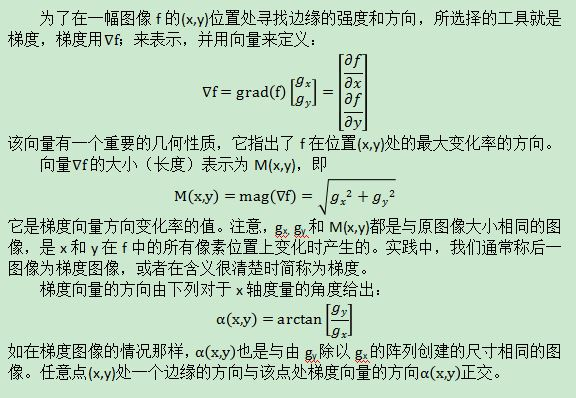### 1.6梯度算子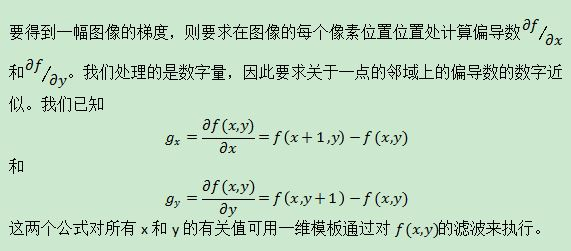#### 1.6.1Roberts算子

当对对角线方向的边缘感兴趣时，我们需要一个二维模板。Roberts算子是最早尝试使用具有对角优势的二维模板之一。Roberts算子是一种最简单的算子，是一种利用局部差分算子寻找边缘的算子，他采用对角线方向相邻两象素之差近似梯度幅值检测边缘。检测垂直边缘的效果好于斜向边缘，定位精度高，对噪声敏感,无法抑制噪声的影响。Roberts边缘算子是一个2x2的模板，采用的是对角方向相邻的两个像素之差。从图像处理的实际效果来看，边缘定位较准，对噪声敏感。
Roberts算子检测方法对具有陡峭的低噪声的图像处理效果较好，但是利用roberts算子提取边缘的结果是边缘比较粗，因此边缘的定位不是很准确。

#### 1.6.2Prewitt算子

Prewitt算子是一种一阶微分算子的边缘检测，利用像素点上下、左右邻点的灰度差，在边缘处达到极值检测边缘，去掉部分伪边缘，对噪声具有平滑作用。其原理是在图像空间利用两个方向模板与图像进行邻域卷积来完成的，这两个方向模板一个检测水平边缘，一个检测垂直边缘。对数字图像f(x，y)，Prewitt算子的定义如下：
G(i)=|[f(i-1,j-1)+f(i-1,j)+f(i-1，j+1)]-[f(i+1,j-1)+f(i+1，j)+f(i+1，j+1)]|
G(j)=|[f(i-1,j+1)+f(i,j+1)+f(i+1，j+1)]-[f(i-1,j-1)+f(i,j-1)+f(i+1，j-1)]|

P(i,j)=max[G(i),G(j)]或 P(i,j)=G(i)+G(j)

#### 1.6.3 Sobel算子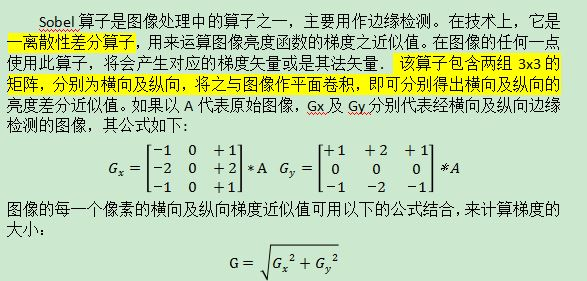Sobel 算子有两个，一个是检测水平边沿的；另一个是检测垂直平边沿的。 Sobel算子另一种形式是各向同性Sobel算子，也有两个，一个是检测水平边沿的，另一个是检测垂直平边沿的。各向同性Sobel算子比普通Sobel算子的位置加权系数更为准确，在检测不同方向的边沿时梯度的+-幅度一致。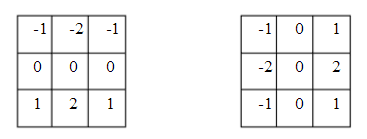Sobel算子认为邻域的像素对当前像素产生的影响不是等价的，所以距离不同的像素具有不同的权值，对算子结果产生的影响也不同。一般来说，距离越大，产生的影响越小。

## 二、算法步骤

Step1.为降噪对图像进行平滑处理，对图像进行高斯滤波。
Step2.用sobel算子进行边缘检测
Step3.用prewitt算子进行边缘检测
Step4.用roberts算子进行边缘检测
Step5.把原图像用拉普拉斯算子的模板处理
Step6.把A图像归零
Step7.把A的R分量用拉普拉斯算子模板处理
Step8.把A的G分量用拉普拉斯算子模板处理
Step9.把A的B分量用拉普拉斯算子模板处理
Step10.令B=I-A

Step11.显示B图像

## 三、结果分析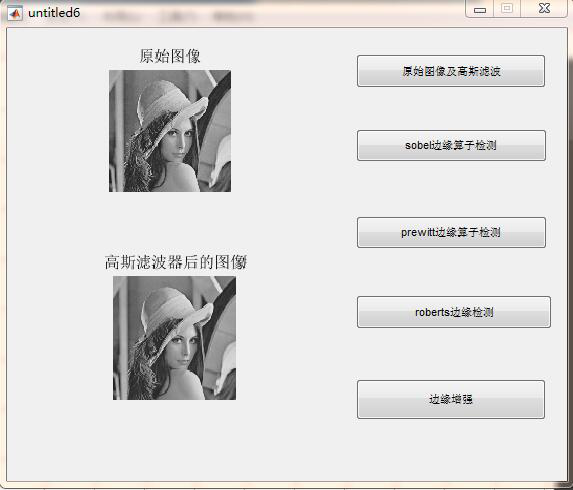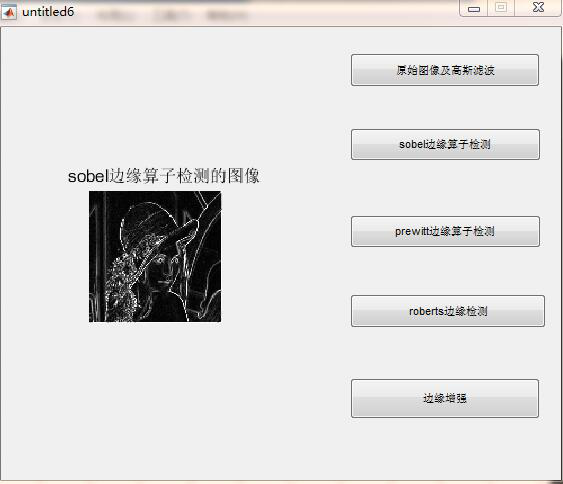Sobel算子并没有将图像的主体与背景严格地区分开来。Sobel算子是一阶微分算子，是加权平均滤波，且检测的图像边缘可能大于两个像素，和prewitt算子一样对灰度渐变低噪声的图像有较好的检测效果，但是对于混合多复杂噪声的图像，处理效果显得不理想。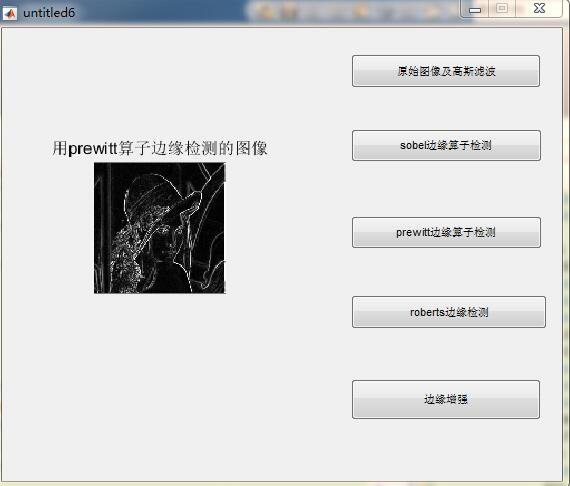Prewitt算子是一阶微分算子，是平均滤波，对灰度渐变低噪声的图像有较好的检测效果。但是，对于混合多复杂噪声的图像，处理效果显得不理想。尽管如此，prewitt模板实现起来仍旧比sobel模板更为简单。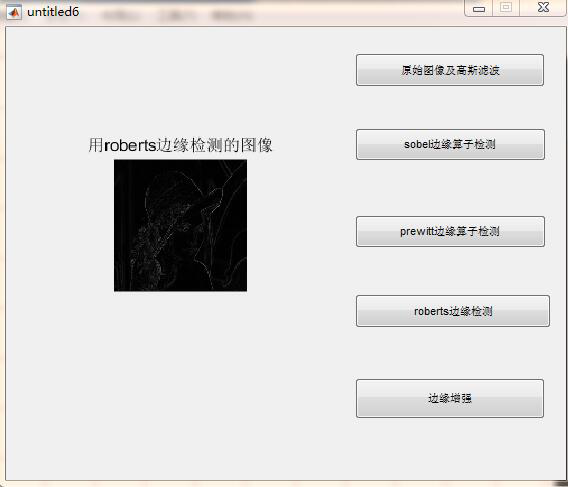Roberts算子检测方法对具有陡峭的低噪声的图像处理效果较好，但是利用roberts算子提取边缘的结果是边缘比较粗，因此边缘的定位不是很准确。由于不包括平滑，所以对噪声比较敏感。## 四、完整程序

```clear all
clc
hg=zeros(3,3);  %设定高斯平滑滤波模板的大小为3*3
delta=0.5;
for x=1:1:3
for y=1:1:3
u=x-2;
v=y-2;
hg(x,y)=exp(-(u^2+v^2)/(2*pi*delta^2));
end
end
h=hg/sum(hg(:));
f=rgb2gray(im2double(g));
subplot(2,3,1);imshow(f);
title('原始图像');
[m,n]=size(f);
ftemp=zeros(m,n);
rowhigh=m-1;
colhigh=n-1;
%%%高斯滤波%%%
for x=2:1:rowhigh-1
for y=2:1:colhigh-1
mod=[f(x-1,y-1) f(x-1,y) f(x-1,y+1); f(x,y-1) f(x,y) f(x,y+1);f(x+1,y-1) f(x+1,y) f(x+1,y+1)];
A=h.*mod;
ftemp(x,y)=sum(A(:));
end
end
f=ftemp;
subplot(2,3,2),imshow(f)
title('高斯滤波器后的图像');
%%%%3*3的sobel算子%%%%%%%%
sx=[-1 -2 -1;0 0 0;1 2 1];
sy=[-1 0 1;-2 0 2;-1 0 1];
for x=2:1:rowhigh-1
for y=2:1:colhigh-1
mod=[f(x-1,y-1) f(x-1,y) f(x-1,y+1); f(x,y-1) f(x,y) f(x,y+1);f(x+1,y-1) f(x+1,y) f(x+1,y+1)];
fsx=sx.*mod;
fsy=sy.*mod;
ftemp(x,y)=max(abs(sum(fsx(:))),abs(sum(fsy(:))));
end
end
fs=im2uint8(ftemp);
subplot(2,3,3),imshow(fs)
title('用sobel边缘算子检测的图像');
% %%%利用prewitt算子进行边缘检测%%%
% %%%%%%%%%%%%%%%%%%%%%%%%%%%%%%%
sx=[-1 -1 -1;0 0 0;1 1 1];
sy=[1 0 -1;1 0 -1;1 0 -1];%这里是prewitt算子

for x=2:1:rowhigh-1
for y=2:1:colhigh-1
mod=[f(x-1,y-1) f(x-1,y) f(x-1,y+1); f(x,y-1) f(x,y) f(x,y+1);f(x+1,y-1) f(x+1,y) f(x+1,y+1)];
fsx=sx.*mod;
fsy=sy.*mod;
ftemp(x,y)=sqrt((sum(fsx(:)))^2+(sum(fsy(:)))^2);
end
end
fr=im2uint8(ftemp);
subplot(2,3,4),imshow(fr)
title('用prewitt算子边缘检测的图像');
% %%%利用roberts算子进行边缘检测%%%
% %%%%%%%%%%%%%%%%%%%%%%%%%%%%%%%
sx=[-1 0;0 1];
sy=[0 -1;1 0];
for x=2:1:rowhigh-1
for y=2:1:colhigh-1
mod=[f(x-1,y-1) f(x-1,y);f(x,y-1) f(x,y)];
fsx=sx.*mod;
fsy=sy.*mod;
ftemp(x,y)=max(abs(sum(fsx(:))),abs(sum(fsy(:))));
end
end
ft=im2uint8(ftemp);
subplot(2,3,6),imshow(ft)
title('用roberts边缘检测的图像');
%拉普拉斯算子锐化图像，用二阶微分
%四邻接g(x,y)=[f(x+1,y)+f(x-1,y)+f(x,y+1)+f(x,y-1)]-4f(x,y)
I=im2double(g);
[m,n,c]=size(I);
A=zeros(m,n,c);
%分别处理R、G、B
%先对R进行处理
for i=2:m-1
for j=2:n-1
A(i,j,1)=I(i+1,j,1)+I(i-1,j,1)+I(i,j+1,1)+I(i,j-1,1)-4*I(i,j,1);
end
end

%再对G进行处理
for i=2:m-1
for j=2:n-1
A(i,j,2)=I(i+1,j,2)+I(i-1,j,2)+I(i,j+1,2)+I(i,j-1,2)-4*I(i,j,2);
end
end

%最后对B进行处理
for i=2:m-1
for j=2:n-1
A(i,j,3)=I(i+1,j,3)+I(i-1,j,3)+I(i,j+1,3)+I(i,j-1,3)-4*I(i,j,3);
end
end
B=I-A;
Figure
imwrite(B,’11.jpg’,’jpg’)
subplot(121)
subplot(122)
imshow('11.jpg');title('边缘增强图像')```